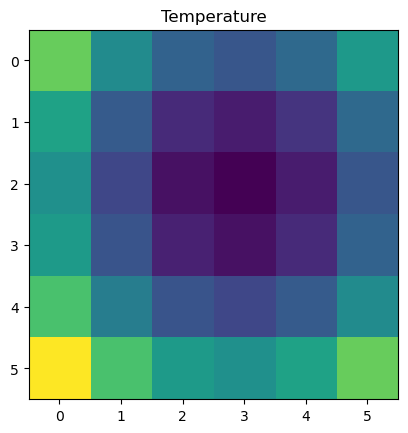Learning Objectives

• Create new raster objects

• Assign the correct projection or CRS

# Spatial Raster Data in Python#

A raster data model uses an array of cells, or pixels, to represent real-world objects. Raster datasets are commonly used for representing and managing imagery, surface temperatures, digital elevation models, and numerous other entities. A raster can be thought of as a special case of an area object where the area is divided into a regular grid of cells. But a regularly spaced array of marked points may be a better analogy since rasters are stored as an array of values where each cell is defined by a single coordinate pair inside of most GIS environments. Implicit in a raster data model is a value associated with each cell or pixel. This is in contrast to a vector model that may or may not have a value associated with the geometric primitive.

In order to work with raster data we will be using rasterio and later geowombat. Behind the scenes a numpy.ndarray does all the heavy lifting. To understand how raster works it helps to construct one from scratch.

Here we create two ndarray objects one X spans [-90°,90°] longitude, and Y covers [-90°,90°] latitude.

import numpy as np
x = np.linspace(-90, 90, 6)
y = np.linspace(90, -90, 6)
X, Y = np.meshgrid(x, y)
X

array([[-90., -54., -18.,  18.,  54.,  90.],
[-90., -54., -18.,  18.,  54.,  90.],
[-90., -54., -18.,  18.,  54.,  90.],
[-90., -54., -18.,  18.,  54.,  90.],
[-90., -54., -18.,  18.,  54.,  90.],
[-90., -54., -18.,  18.,  54.,  90.]])


Let’s generate some data representing temperature and store it an array Z

import matplotlib.pyplot as plt

Z1 =  np.abs(((X - 10) ** 2 + (Y - 10) ** 2) / 1 ** 2)
Z2 =  np.abs(((X + 10) ** 2 + (Y + 10) ** 2) / 2.5 ** 2)
Z =  (Z1 - Z2)

plt.imshow(Z)
plt.title("Temperature")
plt.show()Note that Z contains no data on its location. Its just an array, the information stored in x and y aren’t associated with it at all. This location data will often be stored in the header of file. In order to ‘locate’ the array on the map we will use affine transformations.

## Assigning spatial data to an array in python#

Ok we have an array of data and some coordinates for each cell, but how do we create a spatial dataset from it? In order to do this we need three components:

1. An array of data and typically the xy coordinates

2. A coordinate reference system which defines what coordinate space we are using (e.g. degrees or meters, where is the prime meridian etc)

3. A transform defining the coordinate of the upper left hand corner and the cell resolution

Note

These topic is covered extensively in the next chapter. We will briefly introduce the topic here, but will go into the details later.

Once you have those components you can write out a working spatial raster dataset in python in a few lines of code. We just need to provide the information listed above in a format that rasterio understands.

from rasterio.transform import Affine
import rasterio as rio

res = (x[-1] - x) / 240.0
transform = Affine.translation(x - res / 2, y - res / 2) * Affine.scale(res, res)

# open in 'write' mode, unpack profile info to dst
with rio.open(
"../temp/new_raster.tif",
"w",
driver="GTiff",         # output file type
height=Z.shape,      # shape of array
width=Z.shape,
count=1,                # number of bands
dtype=Z.dtype,          # output datatype
crs="+proj=latlong",    # CRS
transform=transform,    # location and resolution of upper left cell
) as dst:
# check for number of bands
if dst.count == 1:
# write single band
dst.write(Z, 1)
else:
# write each band individually
for band in range(len(Z)):
# write data, band # (starting from 1)
dst.write(Z[band], band + 1)


Note

Raster data is often ‘multiband’ (e.g. red, green, blue), so I provided code that works for both multiband and single band data.

If you are storing multiband data the dimensions should be stored as (band, y, x ).